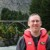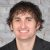# Is It Possible to Have Contradicting Pearson Coefficients and P-values?

Six Sigma – iSixSigma Forums General Forums Methodology Is It Possible to Have Contradicting Pearson Coefficients and P-values?

Viewing 6 posts - 1 through 6 (of 6 total)
• Author
Posts
• #208922Andrew
Participant

For regression, with a simple input X and output Y, Pearson’s coefficient tells us there is a strong linear correlation between an input and an output if close to -1 or +1.
In such cases the p-Value is low (which makes sense).

What happens when there is a non-linear correlation between X and Y, what can I use to characterize / prove correlation? In this case the Pearson coefficient would not be able to be used.

Is it at all possible to have contradicting Pearson coefficient and P-Values (for instance Pearson correlation of 0.1 and p_Value of 0.000)?

0
#208930Robert Butler
Participant

Pearson’s correlation coefficient is only useful in the situation where the relationship between two variables is a straight line. If you have a curvilinear relationship between an X and a Y you can still run it by a Pearson’s correlation coefficient and you will find that correlation coefficient is low because the relationship is no longer a straight line. In a similar manner, if you try to fit a simple Y = X regression line to a curvilinear relationship you will find the p-value is most likely not significant. On the other hand, if you fit a Y = fn of X and X*X then you will have a significant P-value and, depending on the kind of curvilinear relationship you might have a significant p-value for both the linear and the quadratic term in the regression.
Just to check this out try the following simple data set
x1 y1 y2

1 1 1
2 3 2.1
3 5 2.9
4 5.5 4.2
5 6 4.9
6 5.8 6.2
7 5.1 6.8
8 4.9 8
9 4.0 9.2

You will find your Pearson’s correlation coefficient for y1 vs x1 is not significant with a value of .519 and a p = .152. If you run a simple regression of y1 = x1 you will get the same p-value. If you run y1 = fn(x and x*x) you will get p-values of <.0001 for both terms. If you run y2 vs x1 you will get a significant Pearson’s correlation coefficient of .998 and p <.0001 and a similar p-value for the regression of y2 vs x1.

By the way the term linear regression only refers to the linearity of the coefficients in the regression equation. Linear regressions can have polynomial terms. Non-linear refers to non-linear in the regression coefficients and is a very different animal.

0
#209072Andrew
Participant

Thank you very much for the data set, what you said makes sense.
From what I see it is not possible to get contradicting Pearson coefficients and P-Values.
If the Pearson number is high, the P-Value will be low (-> there is a limear correlation)
If the Pearson number is low, the P-Value will be high (-> there is no linear correlation).

After giving it some further thought I would say that the Rsquared adjusted is a better predictor of correlation than Pearson’s correlation factor – as it can be calculated for linear, quadratic and cubic regression models.

0
#209079Robert Butler
Participant

Well, yes/no/maybe. The issue with the simple Pearson coefficient is that you can get “large” values and still have lack of significance. As for Rsquared -no, it is an easily manipulated/fooled statistic and, by itself, tells you very little. There are websites, blogs, even some papers and textbooks that will offer various “rules-of-thumb” with respect to assessing a regression effort on the basis of R2. Usually the rule-of-thumb is that R2 must be some value (.7 is a favorite) or higher before the regression equation is of any value – this is pure rubbish. The question isn’t one of R2, the question is what is the reason for building the regression equation?

One quick example with respect to the absurdity of insisting on given levels of R2: Many years ago I had to analyze the presence of compounds in a water supply which, when present, indicated cyanide leaching. We ran the analysis over time and found a significant positive trend in the data. The R2 for the regression was .04. The crucial fact of the analysis was that the measured concentration of these compounds were increasing over time and, based on that regression, we could show that the trend predicted the cyanide level in the water would reach a life threatening level in about 3+ years time. The analysis wound up as part of a court battle between the local government and the firm responsible for the problem. I wasn’t present at the hearing and the firm dragged in some clown who had citations with respect to the “need” for high R2 before one could accept the results of a regression. End result – the firm won and about 4 years later the level of cyanide leaching reached the critical level. The cost of addressing the problem 4 years later was a lot more than it would have been at the time of the analysis

If you are going to assess a correlation based on a regression then the only proper way to assess the success of that correlation is to do a regression ANALYSIS which means doing all of the plotting and examining of residuals that are at the heart of a regression ANALYSIS. I would recommend you find a good book on regression analysis and memorize the chapter(s) concerning the correct methods for assessing the value of a regression equation. Whatever you do, don’t make the mistake of judging a regression using only summary statistics like Rsquared.

0
#209101Chris Seider
Participant

Look up the “fat pencil” technique and do NOT forget to ALWAYS graphically show the relationship between the X and Y’s you’re looking at.

0
#209365Brion Hurley
Participant

To reinforce these points, check out these two sites

Random correlations that make no sense: http://www.tylervigen.com/spurious-correlations

Many ways to get the same correlation results with different data: https://www.autodeskresearch.com/publications/samestats

First link reinforces correlation does not equal causation, and second link proves you need to look at the data graphically to understand why it is or is not correlated

0
Viewing 6 posts - 1 through 6 (of 6 total)

You must be logged in to reply to this topic.